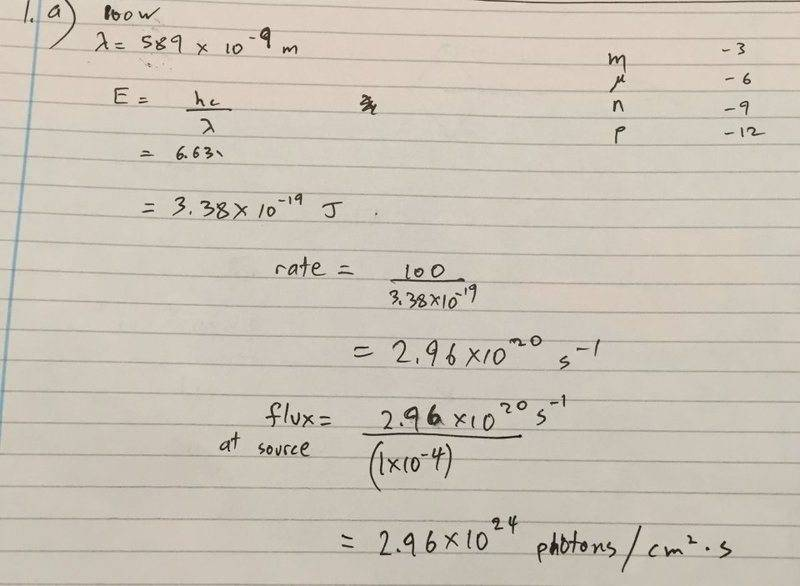# Calculate photon flux at a distance from the source

## Homework Statement

A 100W sodium lamp(lambda=589nm) radiates energy uniformly in all directions.

(A) At what distance from the lamp will a totally absorbing screen absorb photons at the rate of 1.00 photon/cm2.s?
(B) What is the photon flux on a small screen 2m from the lamp?

## Homework Equations

E=hf
photon flux=#of photons/m2.s

## The Attempt at a Solution

I tried relating the area of the absorbing surface to the distance.

gneill
Mentor

## Homework Statement

A 100W sodium lamp(lambda=589nm) radiates energy uniformly in all directions.

(A) At what distance from the lamp will a totally absorbing screen absorb photons at the rate of 1.00 photon/cm2.s?
(B) What is the photon flux on a small screen 2m from the lamp?

## Homework Equations

E=hf
photon flux=#of photons/m2.s

## The Attempt at a Solution

I tried relating the area of the absorbing surface to the distance.
Can you show the details of your attempt?

Can you show the details of your attempt?
This is as far as I got. I tried drawing pyramids with square bases( the source being the tip of the pyramid and the absorbing surface being the square) as well.gneill
Mentor
Okay, your rate of photon production looks good.

The problem states that these photons are radiated in all directions uniformly. What kind of symmetry does that imply (what sort of surface would surround the source uniformly at some constant radial distance)?

Okay, your rate of photon production looks good.

The problem states that these photons are radiated in all directions uniformly. What kind of symmetry does that imply (what sort of surface would surround the source uniformly at some constant radial distance)?

The bulb is inside of a hollow sphere I assume? That being said I can't think of any equations that can relate the distance to a circle of 1cm2 in the sphere.

gneill
Mentor
All you need to know is the rate that photons pass through a spherical surface of radius r. You have the rate of photon production, and you should be able to determine the total surface area of a sphere of radius r. Express it in square centimeters...

All you need to know is the rate that photons pass through a spherical surface of radius r. You have the rate of photon production, and you should be able to determine the total surface area of a sphere of radius r. Express it in square centimeters...
Thanks!! I got the answer which was 4.85x10^7m.

As for the second part, I tried drawing a right-angled triangle with the distance being the longer non-hypotenuse. I'm assuming the screen is a square.

gneill
Mentor
You can use the same method as in part a, only here you're given the radius as 2 meters. Find the flux in photons/cm2, then the geometry of the screen won't matter.

You can use the same method as in part a, only here you're given the radius as 2 meters. Find the flux in photons/cm2, then the geometry of the screen won't matter.
Thanks, I got the answer to that as well.

If you don't mind, I'm curious as to why the geometry doesn't matter though.

Thank you very much for all your help :)

gneill
Mentor
If you don't mind, I'm curious as to why the geometry doesn't matter though.
If the screen is small compared to the radius then its surface is all at approximately the same distance from the source. So all of its surface, regardless of shape, receives the same uniform flux. To be more rigorous one might consider the cross sectional area presented to the source to deal with angled surfaces or shadowed parts, but for a simple small flat surface facing the source, its shape (round, square, rectangular, etc) won't make a difference.

If the screen is small compared to the radius then its surface is all at approximately the same distance from the source. So all of its surface, regardless of shape, receives the same uniform flux. To be more rigorous one might consider the cross sectional area presented to the source to deal with angled surfaces or shadowed parts, but for a simple small flat surface facing the source, its shape (round, square, rectangular, etc) won't make a difference.
I see, thanks! :)